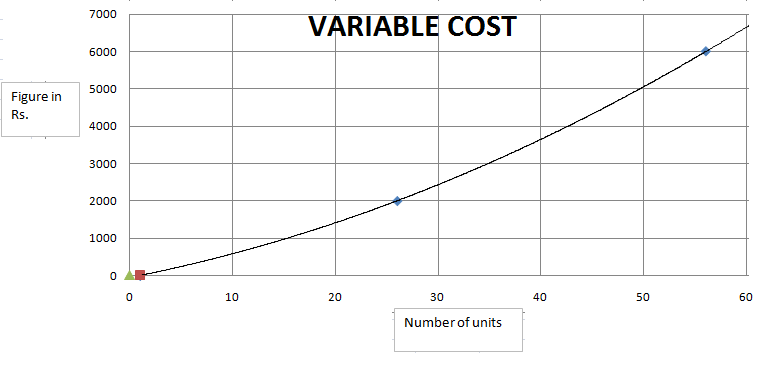### CAT 2000 Question Paper Question 35

Instructions

Answer these questions based on the following Information:

ABC Ltd. produces widgets for which the demand is unlimited and they can sell all of their production. The graph below describes the monthly variable costs incurred by the company as a function of the quantity produced. In addition, operating the plant for one shift results in a fixed monthly cost of Rs. 800. Fixed monthly costs for second shift operation are estimated at Rs. 1200. Each shift operation provides capacity for producing 30 widgets per month.
Note: Average unit cost, AC = Total monthly costs/monthly production, and Marginal cost MC is the rate of change in total cost for unit change in quantity produced.Question 35

# Suppose that each widget sells for Rs. 150. What is the profit earned by ABC Ltd. in July, if it is know that 40 widgets were produced in this month? (Profit is defined as the excess of sales revenue over total cost).

Solution

Total sales = 150 * 40 = 6000. Total costs = 2000 + 3650(approx).= 5600 to 5700 .Hence , profit = 6000 - 5600 = 400 (approx). Hence option C.